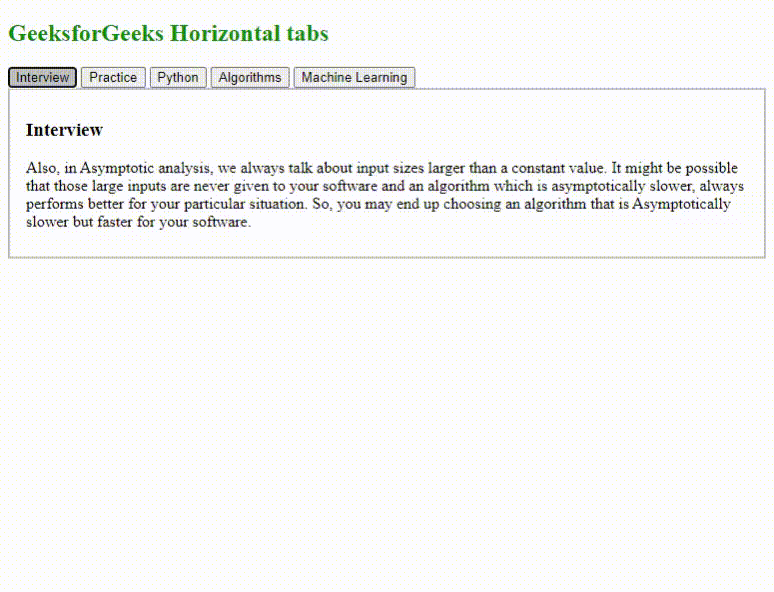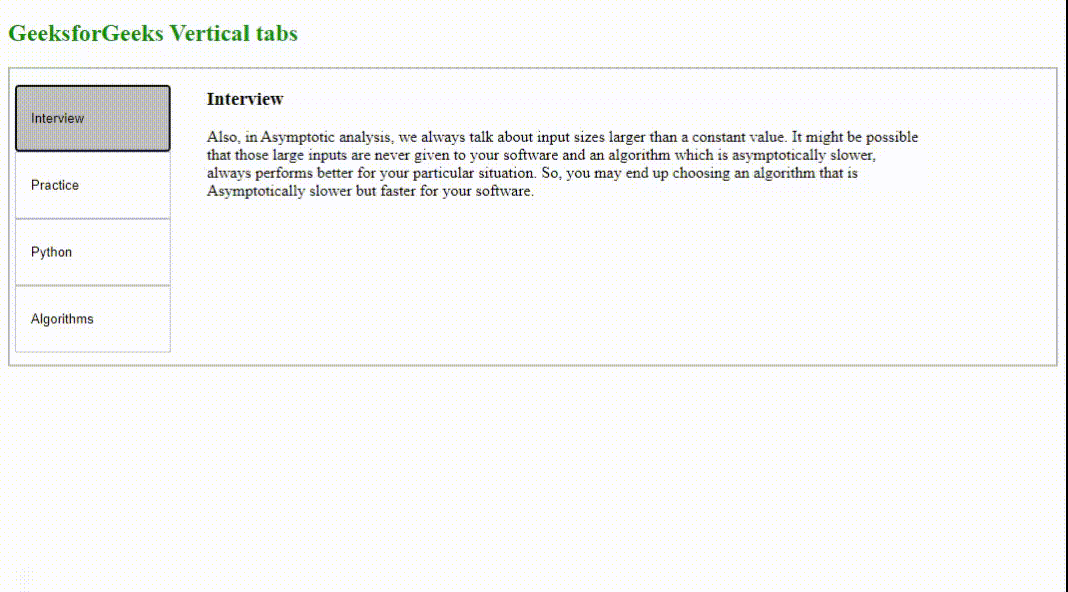Related Articles
How to Create Horizontal and Vertical Tabs using JavaScript ?
• Last Updated : 18 Nov, 2020

Tabs can be used for displaying large amounts of content on a single page in an organized manner. We can design single page tabs using HTML, CSS, and JavaScript. HTML elements are used to design the structure of the tabs and their content in paragraphs. The styling is performed using CSS. On the click of each tab button, the tabs display their respective content. This is done using JavaScript.

Horizontal Tabs: The following code demonstrates the simple HTML structure with tabs and its contents in the form of a paragraph. On click of each tab, it calls the displayContent() method implemented in the “script.js” file given below.

HTML Code:

## HTML

 ` ` `<``html``> ` ` `  `<``head``> ` `    ``<``link` `rel``=``"stylesheet"` `href``=``"style.css"``> ` `    ``<``script` `src``=``"script.js"``> ` ` ` ` `  `<``body``> ` `    ``<``h2` `style``=``"color:green"``> ` `        ``GeeksforGeeks Horizontal tabs ` `    `` ` ` `  `    ``` `    ``<``div` `id``=``"tabsDiv"``> ` `        ``<``button` `class``=``"linkClass"` `onclick``= ` `            ``"displayContent(event, 'interview')"``> ` `            ``Interview ` `        `` ` ` `  `        ``<``button` `class``=``"linkClass"` `onclick``= ` `            ``"displayContent(event, 'practice')"``> ` `            ``Practice ` `        `` ` ` `  `        ``<``button` `class``=``"linkClass"` `onclick``= ` `            ``"displayContent(event, 'python')"``> ` `            ``Python ` `        `` ` ` `  `        ``<``button` `class``=``"linkClass"` `onclick``= ` `            ``"displayContent(event, 'algorithms')"``> ` `            ``Algorithms ` `        `` ` ` `  `        ``<``button` `class``=``"linkClass"` `onclick``= ` `            ``"displayContent(event, 'machine')"``> ` `            ``Machine Learning ` `        `` ` `    `` ` ` `  `    ``` `    ``<``div` `id``=``"interview"` `class``=``"contentClass"``> ` `        ``<``h3``>Interview ` ` `  `        ``<``p``> ` `            ``Also, in Asymptotic analysis, we always ` `            ``talk about input sizes larger than a ` `            ``constant value. It might be possible ` `            ``that those large inputs are never given ` `            ``to your software and an algorithm which is ` `            ``asymptotically slower, always performs ` `            ``better for your particular situation. ` `            ``So, you may end up choosing an algorithm ` `            ``that is Asymptotically slower but faster ` `            ``for your software. ` `        `` ` ` `  `    `` ` ` `  `    ``<``div` `id``=``"practice"` `class``=``"contentClass"``> ` `        ``<``h3``>Practice ` ` `  `        ``<``p``> ` `            ``Asymptotic Analysis is the big idea ` `            ``that handles above issues in analyzing ` `            ``algorithms. In Asymptotic Analysis, ` `            ``we evaluate the performance of an  ` `            ``algorithm in terms of input size (we  ` `            ``don’t measure the actual running time). ` `            ``We calculate, how does the time ` `            ``(or space) taken by an algorithm ` `            ``increases with the input size. ` `        `` ` ` `  `    `` ` ` `  `    ``<``div` `id``=``"python"` `class``=``"contentClass"``> ` `        ``<``h3``>Python ` ` `  `        ``<``p``> ` `            ``Python is a high-level, general-purpose ` `            ``and a very popular programming language. ` `            ``Python programming language (latest Python 3) ` `            ``is being used in web development, Machine ` `            ``Learning applications, along with all ` `            ``cutting edge technology in Software Industry. ` `            ``Python Programming Language is very well ` `            ``suited for Beginners, also for experienced ` `            ``programmers with other programming languages ` `            ``like C++ and Java. ` `        `` ` `    `` ` ` `  `    ``<``div` `id``=``"algorithms"` `class``=``"contentClass"``> ` `        ``<``h3``>Greedy Algorithms ` ` `  `        ``<``p``> ` `            ``Greedy is an algorithmic paradigm that ` `            ``builds up a solution piece by piece, ` `            ``always choosing the next piece that ` `            ``offers the most obvious and immediate ` `            ``benefit. So the problems where ` `            ``choosing locally optimal also ` `            ``leads to global solution are ` `            ``best fit for Greedy. ` `        `` ` `    `` ` ` `  `    ``<``div` `id``=``"machine"` `class``=``"contentClass"``> ` `        ``<``h3``>Machine Learning ` ` `  `        ``<``p``> ` `            ``Machine Learning is the field of ` `            ``study that gives computers the capability ` `            ``to learn without being explicitly ` `            ``programmed. ML is one of the most ` `            ``exciting technologies that one ` `            ``would have ever come across. ` `            ``As it is evident from the name, ` `            ``it gives the computer that makes ` `            ``it more similar to humans: ` `            ``The ability to learn. Machine learning ` `            ``is actively being used today, ` `            ``perhaps in many more places than ` `            ``one would expect. ` `        `` ` `    `` ` ` ` ` `  ` `

CSS Code: The following code is of the “style.css” file that is used for the styling of the page and the tabs.

## css

 ` `

JavaScript Code: The following code contains the functionality of tabs using JavaScript.

## Javascript

 `function` `displayContent(event, contentNameID) { ` ` `  `    ``let content = ` `        ``document.getElementsByClassName(``"contentClass"``); ` `    ``let totalCount = content.length; ` ` `  `    ``// Loop through the content class ` `    ``// and hide the tabs first ` `    ``for` `(let count = 0; ` `        ``count < totalCount; count++) { ` `        ``content[count].style.display = ``"none"``; ` `    ``} ` ` `  `    ``let links = ` `        ``document.getElementsByClassName(``"linkClass"``); ` `    ``totalLinks = links.length; ` ` `  `    ``// Loop through the links and ` `    ``// remove the active class ` `    ``for` `(let count = 0; ` `        ``count < totalLinks; count++) { ` `        ``links[count].classList.remove(``"active"``); ` `    ``} ` ` `  `    ``// Display the current tab ` `    ``document.getElementById(contentNameID) ` `        ``.style.display = ``"block"``; ` ` `  `    ``// Add the active class to the current tab ` `    ``event.currentTarget.classList.add(``"active"``); ` `} `

Output:Vertical Tabs: The tabs can be made vertical by changing the HTML structure and replacing the CSS stylesheet with a modified one used for the vertical tabs design. The following code demonstrates the vertical tabs.

HTML code:

## HTML

 ` ` `<``html``> ` ` `  `<``head``> ` `    ``<``link` `rel``=``"stylesheet"` `href``=``"vstyle.css"``> ` `    ``<``script` `src``=``"script.js"``> ` ` ` ` `  `<``body``> ` `    ``<``h2` `style``=``"color:green"``> ` `        ``GeeksforGeeks Vertical tabs ` `    `` ` ` `  `    ``<``div` `id``=``"tabsDiv"``> ` `        ``<``div` `id``=``"interview"` `class``=``"contentClass"``> ` `            ``<``h3``>Interview ` ` `  `            ``<``p``> ` `                ``Also, in Asymptotic analysis, ` `                ``we always talk about input sizes larger ` `                ``than a constant value. It might ` `                ``be possible that those large inputs ` `                ``are never given to your software and ` `                ``an algorithm which is asymptotically ` `                ``slower, always performs better for ` `                ``your particular situation. So, you ` `                ``may end up choosing an algorithm that ` `                ``is Asymptotically slower but faster ` `                ``for your software. ` `            `` ` `        `` ` ` `  `        ``<``div` `id``=``"practice"` `class``=``"contentClass"``> ` `            ``<``h3``>Practice ` ` `  `            ``<``p``> ` `                ``Asymptotic Analysis is the big idea ` `                ``that handles above issues in analyzing ` `                ``algorithms. In Asymptotic Analysis, we ` `                ``evaluate the performance of an algorithm ` `                ``in terms of input size (we don’t measure ` `                ``the actual running time). We calculate, ` `                ``how does the time (or space) taken by ` `                ``an algorithm increases with ` `                ``the input size. ` `            `` ` `        `` ` ` `  `        ``<``div` `id``=``"python"` `class``=``"contentClass"``> ` `            ``<``h3``>Python ` ` `  `            ``<``p``> ` `                ``Python is a high-level, general-purpose ` `                ``and a very popular programming language. ` `                ``Python programming language (latest Python 3) ` `                ``is being used in web development, Machine ` `                ``Learning applications, along with all ` `                ``cutting edge technology in Software Industry. ` `                ``Python Programming Language is very ` `                ``well suited for Beginners, also for ` `                ``experienced programmers with other ` `                ``programming languages like C++ and Java. ` `            `` ` `        `` ` ` `  `        ``<``div` `id``=``"algorithms"` `class``=``"contentClass"``> ` `            ``<``h3``>Greedy Algorithms ` ` `  `            ``<``p``> ` `                ``Greedy is an algorithmic paradigm ` `                ``that builds up a solution piece by ` `                ``piece,always choosing the next piece ` `                ``that offers the most obvious and ` `                ``immediate benefit. So the problems ` `                ``where choosing locally optimal also ` `                ``leads to global solution are best ` `                ``fit for Greedy. ` `            `` ` `        `` ` ` `  `        ``<``ul` `class``=``"ulClass"` `style``=``"height:300px"``> ` `            ``<``li` `style``=``"list-style-type:none;"``> ` `                ``<``button` `class``=``"linkClass"` `onclick``= ` `                    ``"displayContent(event, 'interview')"``> ` `                    ``Interview ` `                `` ` `            `` ` `             `  `            ``<``li` `style``=``"list-style-type:none;"``> ` `                ``<``button` `class``=``"linkClass"` `onclick``= ` `                    ``"displayContent(event, 'practice')"``> ` `                    ``Practice ` `                `` ` `            `` ` ` `  `            ``<``li` `style``=``"list-style-type:none;"``> ` `                ``<``button` `class``=``"linkClass"` `onclick``= ` `                    ``"displayContent(event, 'python')"``> ` `                    ``Python ` `                `` ` `            `` ` ` `  `            ``<``li` `style``=``"list-style-type:none;"``> ` `                ``<``button` `class``=``"linkClass"` `onclick``= ` `                    ``"displayContent(event, 'algorithms')"``> ` `                    ``Algorithms ` `                `` ` `            `` ` `        `` ` `    `` ` ` ` ` `  ` `

CSS Code: The below code is the modified CSS code for the vertical tabs display.

## css

 ` `

Output:My Personal Notes arrow_drop_up
Recommended Articles
Page :